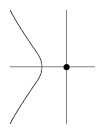# Acnode

Jump to: navigation, search

An older term, hardly used nowadays (2000), for an isolated point, or hermit point, of a plane algebraic curve (cf. also Algebraic curve).Figure: a130100a

For instance, the point $(0,0)$ is an acnode of the curve $X^3+X^2+Y^2=0$ in $\mathbf R^2$.